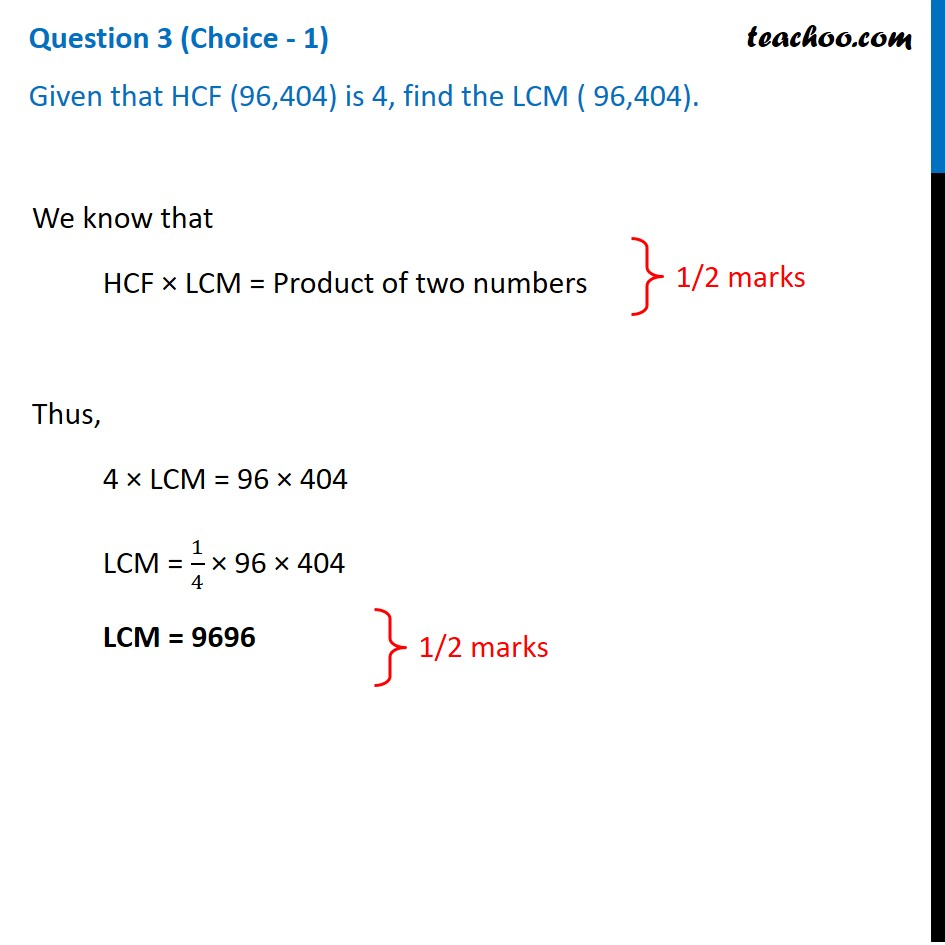CBSE Class 10 Sample Paper for 2021 Boards - Maths Basic

Class 10
Solutions of Sample Papers for Class 10 Boards

## Given that HCF (96,404) is 4, find the LCM ( 96,404).Learn in your speed, with individual attention - Teachoo Maths 1-on-1 Class

### Transcript

Question 3 (Choice - 1) Given that HCF (96,404) is 4, find the LCM ( 96,404).We know that HCF × LCM = Product of two numbers Thus, 4 × LCM = 96 × 404 LCM = 1/4 × 96 × 404 LCM = 9696 1/2 marks 1/2 marks